• 二阶混合偏导数顺序
千次阅读
2019-09-17 11:07:19

正确的结论应为：

二阶混合偏导数在“(二阶混合偏导数)连续”的条件下与求导的次序无关

参考文章：二阶混合偏导数在连续的条件下与求导的次序无关 A. 错误 B. 正确

更多相关内容
• 二阶混合偏导 文章目录二阶混合偏导wiki武汉大学同济大学总结 偏导数是多元函数求导过程中的一个概念。 这里主要阐明一个事实：中国教材和外国教材在二阶混合偏导的记法上是有差别的。 外国： 先求导的变量写...

# 二阶混合偏导

### 文章目录

偏导数是多元函数求导过程中的一个概念。

这里主要阐明一个事实：中国教材和外国教材在二阶混合偏导的记法上是有差别的。

外国：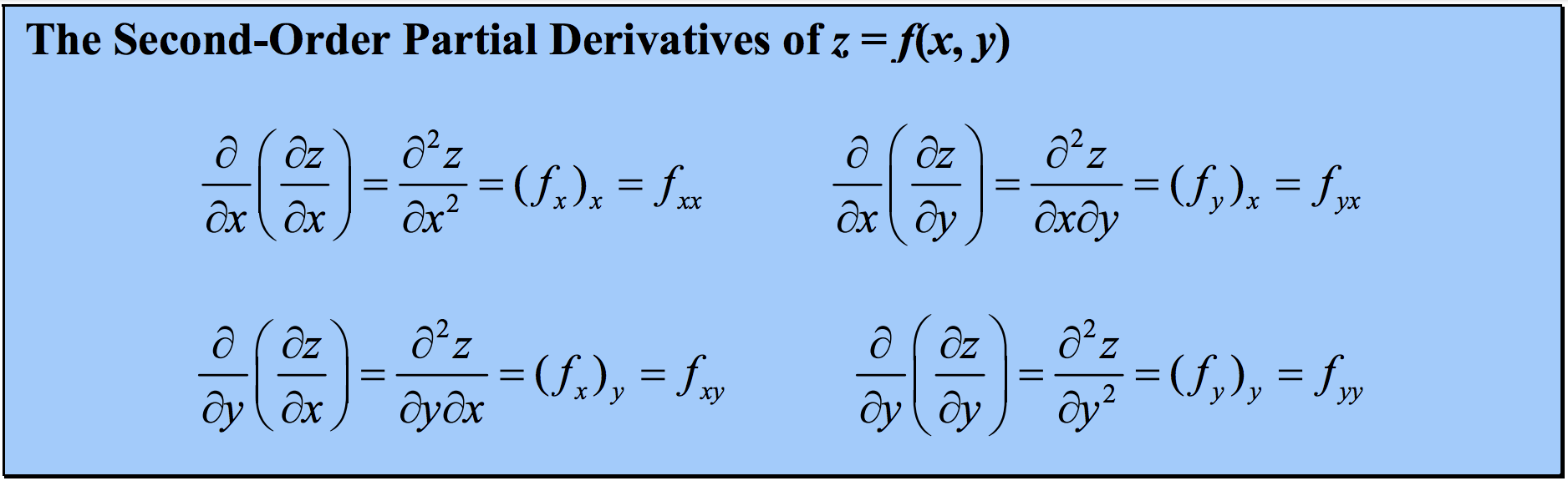先求导的变量写在后面。

这种记法是国际上公认的记法，包括wiki.

## wiki

wiki上关于二阶混合偏导的记法如下：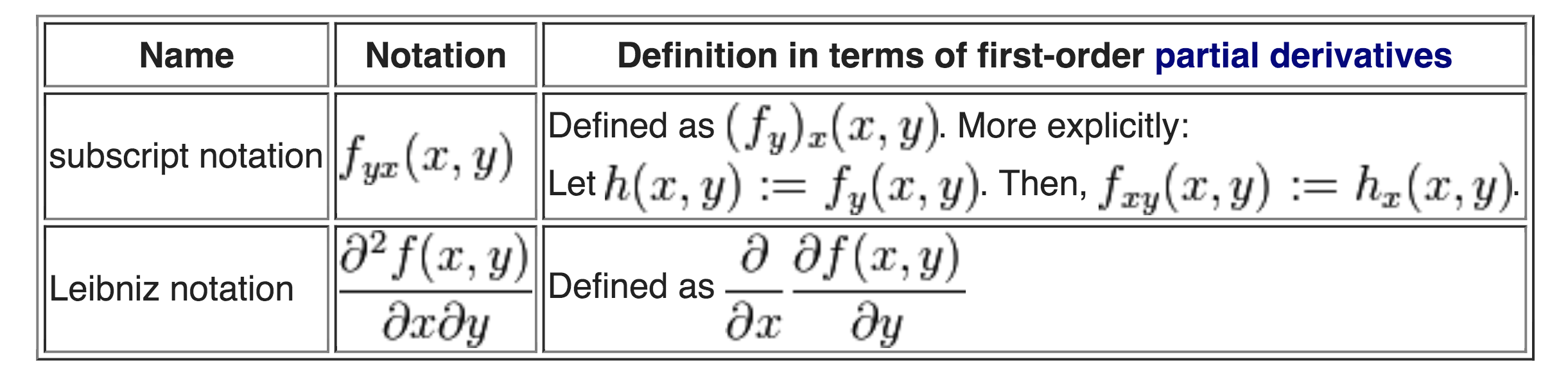然而，我在同济大学《高等数学》第七版下册和武汉大学《高等数学》下册上看到的记法是相反的。

## 武汉大学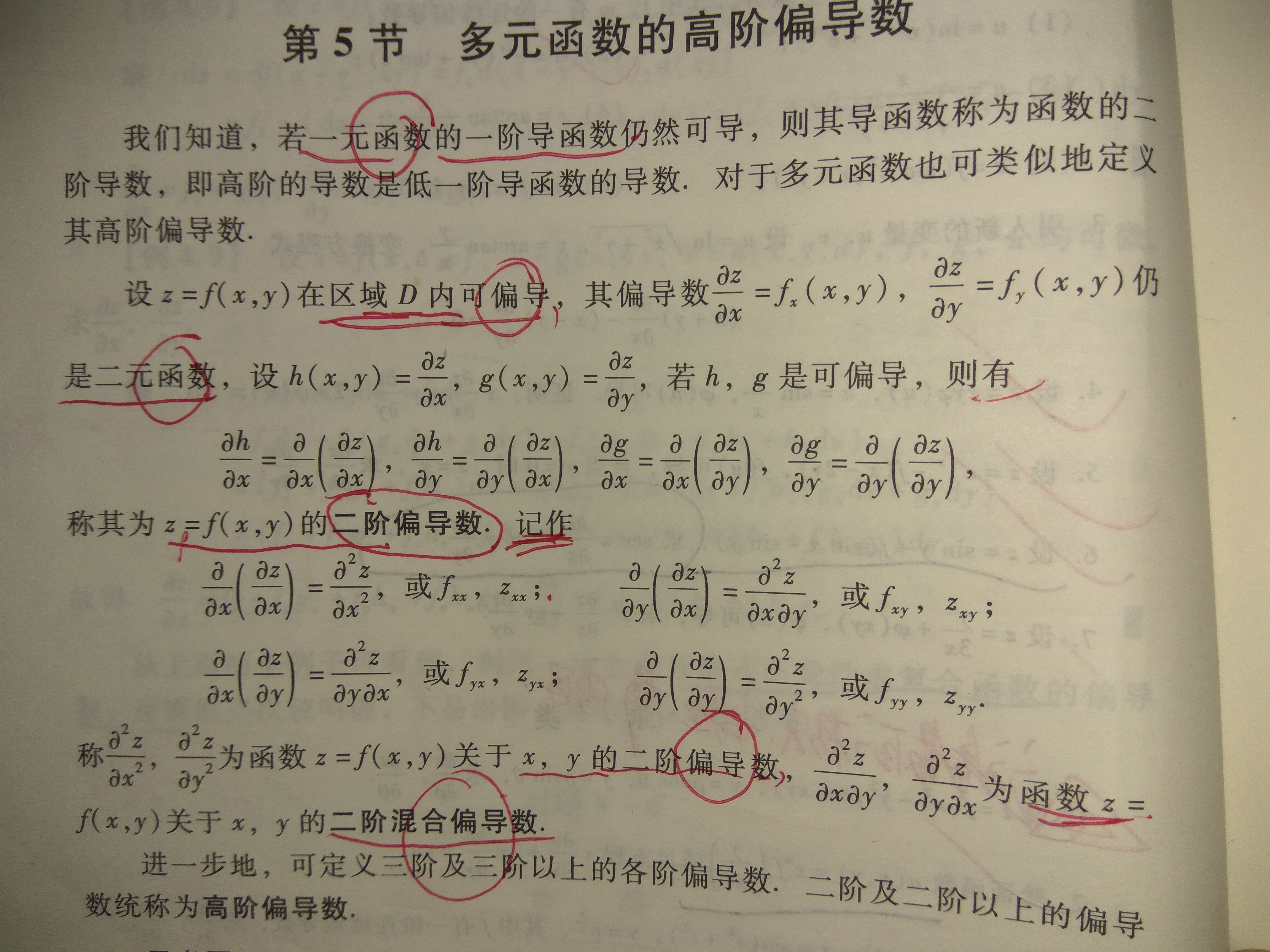可见，先求导的变量写在前面。

## 同济大学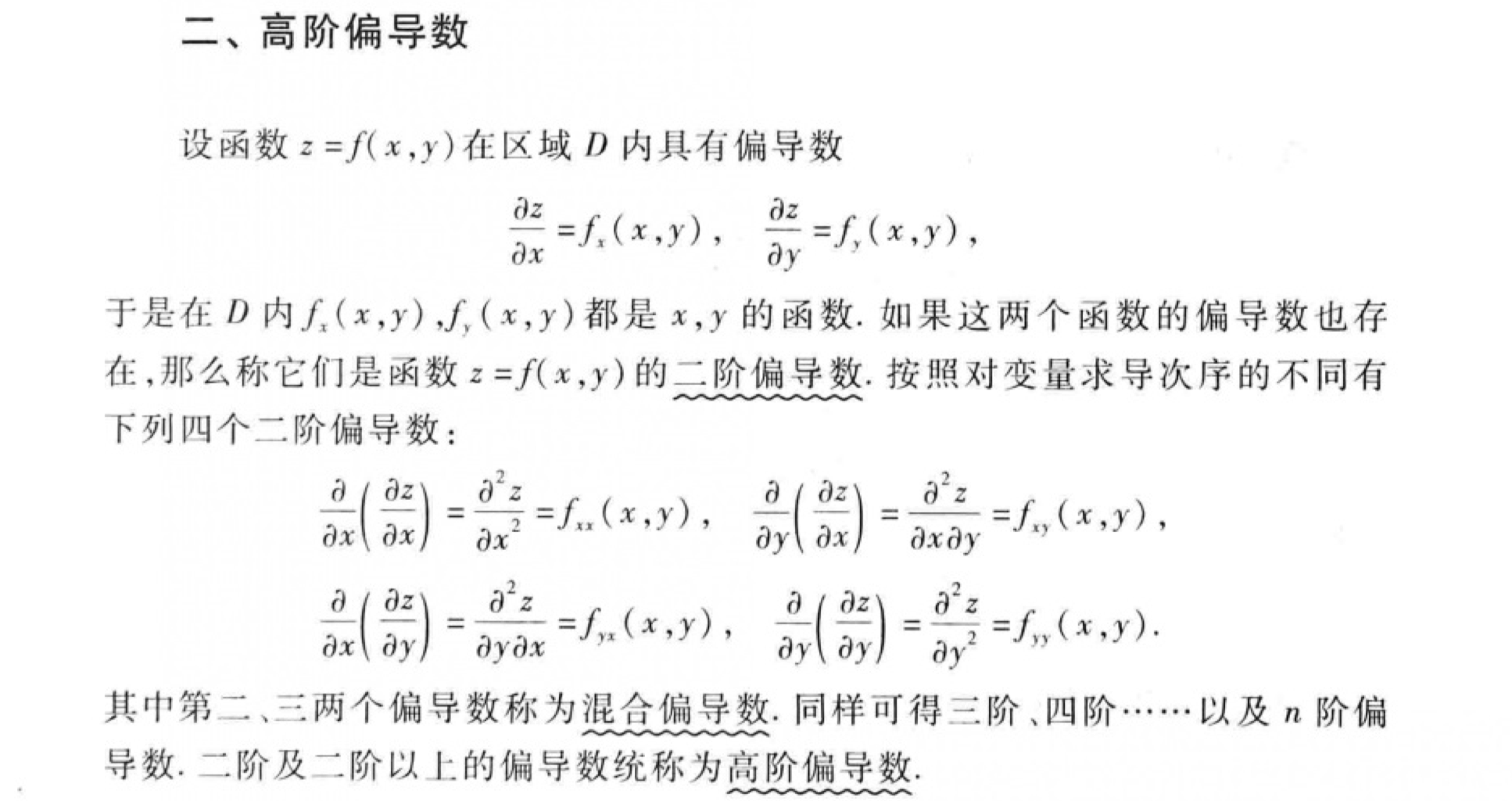可见，先求导的变量写在前面。

## 总结

国际(wiki)上的二阶混合偏导，先求导的变量写在后面。
国内（至少是武大和同济)，先求导的变量写在前面。
所以，对于这一点，我们一定要做到心里有数。
好在还有一个定理，让我们不必总是很忧虑。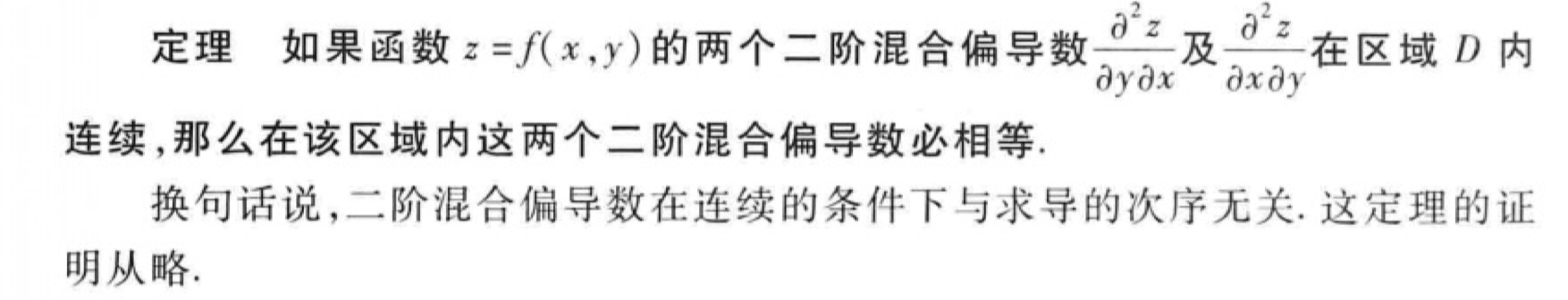即二阶混合偏导数在连续的条件下与求导的次序无关。

展开全文• 海森矩阵的混合偏导数是海森矩阵非主对角线上的元素。假如他们是连续的，那么求导顺序没有区别 ，即 上式也可写为 在正式写法中， 如果  f  函数在区域  D  内连续并处处存在二阶导数，那么 ...

In mathematics, the Hessian matrix is the square matrix of second-order partial derivatives of afunction; that is, it describes the local curvature of a function of many variables. The Hessian matrix was developed in the 19th century by the German mathematician Ludwig Otto Hesse and later named after him. Hesse himself had used the term "functional determinants".

海森矩阵就是二阶偏导函数的方阵.他描述了局部的曲率函数.

Given the real-valued functionif all second partial derivatives of f exist, then the Hessian matrix of f is the matrixwhere x = (x1x2, ..., xn) and Di is the differentiation operator with respect to the ith argument and the Hessian becomesSome mathematicians define the Hessian as the determinant of the above matrix.

Hessian matrices are used in large-scale optimization problems within Newton-type methods because they are the coefficient of the quadratic term of a local Taylor expansion of a function. That is,where J is the Jacobian matrix, which is a vector (the gradient) for scalar-valued functions. The full Hessian matrix can be difficult to compute in practice; in such situations, quasi-Newtonalgorithms have been developed that use approximations to the Hessian. The most well-known quasi-Newton algorithm is the BFGS algorithm.

在数学中，海森矩阵（Hessian matrix 或 Hessian）是一个自变量为向量的实值函数的二阶偏导数组成的方块矩阵，此函数如下：如果 所有的二阶导数都存在，那么 的海森矩阵即：

H(f)ij(xDiDjf(x)

其中，即可见，多元函数的二阶导数就是一个海森矩阵
海森矩阵被应用于牛顿法解决的大规模优化问题。

混合偏导数和海森矩阵的对称性

海森矩阵的混合偏导数是海森矩阵非主对角线上的元素。假如他们是连续的，那么求导顺序没有区别，即上式也可写为在正式写法中，如果 函数在区域 内连续并处处存在二阶导数，那么 f的海森矩阵在 区域内为对称矩阵。

给定二阶导数连续的函数，海森矩阵的行列式，可用于分辨 的临界点是属于鞍点还是极值点。

对于 的临界点 (x0,y0) 一点，有，然而凭一阶导数不能判断它是鞍点、局部极大点还是局部极小点。海森矩阵可能解答这个问题。：若，则(x0,y0)是局部极小点；若，则(x0,y0)是局部极大点。
：(x0,y0)是鞍点。
：二阶导数无法判断该临界点的性质，得从更高阶的导数以泰勒公式考虑。

MATLAB中获得Hessian矩阵：

The Hessian of scalar valued function f:Rn

首先类比一下一维。Jacobian相当于一阶导数，Hessian相当于二阶导数。 一维函数的导数的motivation是很明显的。二阶导数的零点就是一阶导数的极值点。 对于很多应用，我们不仅关心一阶导数的零点，也关心一阶导数的极值点，比如信号处理中，信号的一阶导数的极值点反映信号变化的最剧烈程度。极值点寻求在编程时不方便，不如找二阶导数的零点。
对于向量场F: Rn-> Rm, Jacobian的每一行实际都是一个梯度。且有 F（X)=F(P)+J(P)(X-P)+O(||X-P||) 这个式子的每一行都是一个分量的局部线性化。
考虑一个二维的数字图像线性变换（Homography， image warping), 以有限差分代替微分，可作类似分析。
H: 像素（x,y)-->像素(u,v)
u=u（x,y) v=v(x,y)
则其Jacobian为
u'(x) u'(y)]
v'(x) v'(y)]
反映了局部图像的变形程度。
最理想的情况 u'(x)=1,v'(y)=1,u'(y)=0,v'(x)=0.说明图像维持原状。
由于 dudv=|det(Jacobian(x,y))|dxdy （此式的有效性可参考换元法）
[注：]有的书上称det(Jacobian(x,y)）为Jacobian.

说明面积微元改变的程度由|det(Jacobian(x,y))|决定
当|det(Jacobian(x,y))|=1时，说明面积不变，
当|det(Jacobian(x,y))|<1时，说明面积压缩，出现了像素丢失现象。
当|det(Jacobian(x,y))|>1时，说明面积扩张，需要进行像素插值。
另外，由Jacobian矩阵的特征值或奇异值，可作类似说明。可参考Wielandt-Hoffman定理

Hessian矩阵定义在标量函数上，对于矢量函数，则成为一个rank 3的张量。

展开全文数学 图像处理算法
• 大学数学 第八章第二节 偏导数 高阶偏导数 概念 用法与实例
• 偏导数

## §第七章第二节偏导数 $\color{blue}{\S 第七章 第二节 偏导数}$

一、偏导数概念及其计算
二、高阶偏导数

### 一、偏导数概念及其计算法 $\color{blue}{一、偏导数概念及其计算法}$

1.z=f(x,y)(x0,y0)limΔx0f(x0+Δx,y0)f(x0,y0)Δx,z=f(x,y)(x0,y0)x,zx(x0,y0);fx(x0,y0);zx|(x0,y0);fx(x0,y0);f1(x0,y0). $定义1.设函数z = f(x, y)在点(x_0, y_0)的某邻域内极限\\ \lim_{\Delta{x} \rightarrow 0}{\dfrac{f(x_0 + \Delta{x}, y_0) - f(x_0, y_0)}{\Delta{x}}} 存在,则称此极限为函数\\ z = f(x, y)在点(x_0, y_0)对x的偏导数,记为\\ \left. \dfrac{\partial z}{\partial x} \right|_ {(x_0, y_0)}; \left. \dfrac{\partial f}{\partial x} \right|_ {(x_0, y_0)}; \left. z_x \right|_ {(x_0, y_0)}; f_x(x_0, y_0); f_1^{\prime}(x_0, y_0).$

yfy(x0,y0)=limΔy0f(x0,y0+Δy)f(x0,y0)Δy=ddyf(x0,y)y=y0 $同样可以定义对y的偏导数\\ f_y(x_0, y_0) = \lim_{\Delta{y} \rightarrow 0}{\dfrac{f(x_0, y_0 + \Delta{y}) - f(x_0, y_0)}{\Delta{y}}} \\ = \left. \dfrac{d}{dy}f(x_0, y) \right|_ {y = y_0}$
z=f(x,y)D(x,y)xy,,,zxfx,zx,fx(x,y),f1(x,y)zyfy,zy,fy(x,y),f2(x,y) $若函数z = f(x, y)在域D内每一点(x, y)处对于x或y偏导数存在,\\ 则该偏导数称为偏导函数,也简称偏导数,记作\\ \dfrac{\partial z}{\partial x}， \dfrac{\partial f}{\partial x}, z_x, f_x(x, y), f_1^{\prime}(x, y) \\ \dfrac{\partial z}{\partial y}， \dfrac{\partial f}{\partial y}, z_y, f_y(x, y), f_2^{\prime}(x, y)$

广. $偏导数的概念可以推广到二元以上的函数.$
,u=f(x,y,z)(x,y,z)xfx(x,y,z)=limΔx0f(x+Δx,y,z)f(x,y,z)Δxfy(x,y,z)=limΔy0f(x,y+Δy,z)f(x,y,z)Δyfz(x,y,z)=limΔz0f(x,y,z+Δz)f(x,y,z)Δz $例如,三元函数u = f(x, y, z)在点(x, y, z)处对x的偏导数定义为\\ f_x(x, y, z) = \lim_{\Delta{x} \rightarrow 0}{\dfrac{f(x + \Delta{x}, y, z) - f(x, y, z)}{\Delta{x}}} \\ f_y(x, y, z) = \lim_{\Delta{y} \rightarrow 0}{\dfrac{f(x , y+ \Delta{y}, z) - f(x, y, z)}{\Delta{y}}} \\ f_z(x, y, z) = \lim_{\Delta{z} \rightarrow 0}{\dfrac{f(x , y, z+ \Delta{z}) - f(x, y, z)}{\Delta{z}}}$

1.z=x2+3xy+y2(1,2). $例1.求z = x^2 + 3xy + y^2在点(1, 2)处的偏导数.$
:1zx=2x+3yzy=3x+2yzx(1,2)=21+32=8zy(1,2)=31+22=72zx(1,2)=limΔx0f(1+Δx,2)f(1,2)Δx=limΔx0[(1+Δx)2+3(1+Δx)2+22][12+312+22]Δx=limΔx0(8+Δx)=8zy(1,2)=limΔy0f(1,2+Δy)f(1,2)Δy=limΔy0[12+31(2+Δy)+(2+Δy)2][12+312+22]Δy=limΔx0(7+Δy)=7 $解:\\ 解法1\\ \dfrac{\partial z}{\partial x} = 2x + 3y \\ \dfrac{\partial z}{\partial y} = 3x + 2y \\ \left. \dfrac{\partial z}{\partial x} \right|_ {(1, 2)} = 2 \cdot 1 + 3 \cdot 2 = 8 \\ \left. \dfrac{\partial z}{\partial y} \right|_ {(1, 2)} = 3 \cdot 1 + 2 \cdot 2 = 7 \\ 解法2：\\ \left. \dfrac{\partial z}{\partial x} \right|_ {(1, 2)} = \lim_{\Delta{x} \rightarrow 0}{\dfrac{f(1 + \Delta{x}, 2) - f(1, 2)}{\Delta{x}}} \\ = \lim_{\Delta{x} \rightarrow 0}{\dfrac{[(1 + \Delta{x})^2 + 3 \cdot (1 + \Delta{x}) \cdot 2 + 2^2] - [1^2 + 3 \cdot 1 \cdot 2 + 2^2]}{\Delta{x}}} \\ = \lim_{\Delta{x} \rightarrow 0}{(8 + \Delta{x})} \\ = 8 \\ \left. \dfrac{\partial z}{\partial y} \right|_ {(1, 2)} = \lim_{\Delta{y} \rightarrow 0}{\dfrac{f(1, 2 + \Delta{y}) - f(1, 2)}{\Delta{y}}} \\ = \lim_{\Delta{y} \rightarrow 0}{\dfrac{[1^2 + 3\cdot1\cdot(2+ \Delta{y}) + (2 + \Delta{y})^2] - [1^2 + 3 \cdot 1 \cdot 2 + 2^2]}{\Delta{y}}} \\ = \lim_{\Delta{x} \rightarrow 0}{(7 + \Delta{y})} \\ = 7$

2.z=xy(x>0,x1),xyzx+1lnxzy=2z $例2.设z = x^y (x > 0, 且 x \neq 1),求证\dfrac{x}{y} \dfrac{\partial z}{\partial x} + \dfrac{1}{\ln{x}} \dfrac{\partial z}{\partial y} = 2z$
:zx=yxy1;zy=xylnxxyzx+1lnxzy=xyyxy1+1lnxxylnx=xy+xy=2z $证:\\ \because \dfrac{\partial z}{\partial x} = yx^{y - 1}; \dfrac{\partial z}{\partial y} = x^y \ln{x}\\ \dfrac{x}{y} \dfrac{\partial z}{\partial x} + \dfrac{1}{\ln{x}} \dfrac{\partial z}{\partial y} \\ = \dfrac{x}{y} \cdot yx^{y -1} + \dfrac{1}{\ln{x}} \cdot x^y\cdot \ln{x} \\ = x^y + x^y \\ = 2z$

3.z=xy+x3,zx+zy $例3.设z = xy + x^3,求\dfrac{\partial z}{\partial x} + \dfrac{\partial z}{\partial y}$
:zx=y+3x2zy=xzx+zy=3x2+x+y $解:\\ \dfrac{\partial z}{\partial x} = y + 3x^2 \\ \dfrac{\partial z}{\partial y} = x \\ \dfrac{\partial z}{\partial x} + \dfrac{\partial z}{\partial y} = 3x^2 + x + y$

4.f(x,y)=sinx2y,fx(x,y),fy(x,y). $例4.设f(x, y) = \sin{x^2y},求f_x^{\prime}(x, y),f_y^{\prime}(x, y).$
:fx(x,y)=(sinx2y)x=cosx2y2xy=2xycosx2yfy(x,y)=(sinx2y)y=cosx2yx2=x2cosx2y $解:\\ f_x^{\prime}(x, y) = (\sin{x^2y}) _x^{\prime} = \cos{x^2y} \cdot 2xy = 2xy \cos{x^2y} \\ f_y^{\prime}(x, y) = (\sin{x^2y}) _y^{\prime} = \cos{x^2y} \cdot x^2 = x^2 \cos{x^2y}$

### 二、高阶偏导数 $\color{blue}{二、高阶偏导数}$

z=f(x,y)Dzx=fx(x,y),zy=fy(x,y),z=f(x,y).,: $设z = f(x, y)在域D内存在连续的偏导数\\ \dfrac{\partial z}{\partial x} = f_x(x, y), \dfrac{\partial z}{\partial y} = f_y(x, y) \\ 若这两个偏导数仍存在偏导数,则称它们是\\ z = f(x, y)的二阶偏导数.按求导顺序不同,有\\ 下列四个二阶偏导数:$
x(zx)=2zx2=fxx(x,y);y(zx)=2zxy=fxy(x,y);x(zy)=2zyx=fyx(x,y);y(zy)=2zy2=fyy(x,y); $\dfrac{\partial }{\partial x}(\dfrac{\partial z }{\partial x}) = \dfrac{\partial ^2 z}{\partial x^2} = f_{xx}(x, y); \\ \dfrac{\partial }{\partial y}(\dfrac{\partial z }{\partial x}) = \dfrac{\partial ^2 z}{\partial x \partial y} = f_{xy}(x, y); \\ \dfrac{\partial }{\partial x}(\dfrac{\partial z }{\partial y}) = \dfrac{\partial ^2 z}{\partial y \partial x} = f_{yx}(x, y); \\ \dfrac{\partial }{\partial y}(\dfrac{\partial z }{\partial y}) = \dfrac{\partial ^2 z}{\partial y^2} = f_{yy}(x, y);$

$类似可以定义更高阶的偏导数$
,z=f(x,y)xx(2zx2)=3zx3 $例如,z = f(x, y)关于x的三阶偏导数为\\ \dfrac{\partial }{\partial x}(\dfrac{\partial ^2 z}{\partial x^2}) = \dfrac{\partial ^3 z}{\partial x^3}$

z=f(x,y)xn1,yy(n1zxn1)=nzxn1y $z = f(x, y)关于x的n - 1阶偏导数,再关于y的一阶偏导数为\\ \dfrac{\partial }{\partial y}(\dfrac{\partial ^{n - 1} z}{\partial x^{n -1}}) = \dfrac{\partial ^n z}{\partial x^{n - 1} \partial y}$

1.z=ex+2y3zyx2 $例1.求函数z = e^{x + 2y}的二阶偏导数及\dfrac{\partial ^3 z}{\partial y \partial x^2}$
zx=ex+2yzy=2ex+2y2zx2=(ex+2y)x=ex+2y2zxy=(ex+2y)y=2ex+2y2zyx=(2ex+2y)x=2ex+2y2zy2=(2ex+2y)y=4ex+2y3zyx2=x(2zyx)=2ex+2y $解：\\ \dfrac{\partial z}{\partial x} = e^{x + 2y} \\ \dfrac{\partial z}{\partial y} = 2e^{x + 2y} \\ \dfrac{\partial^2 z}{\partial x^2} = (e^{x + 2y})_x^{\prime} = e^{x + 2y}\\ \dfrac{\partial ^2 z}{\partial x \partial y} = (e^{x + 2y})_y^{\prime} = 2e^{x + 2y} \\ \dfrac{\partial ^2 z}{\partial y \partial x} = (2e^{x + 2y})_x^{\prime} = 2e^{x + 2y} \\ \dfrac{\partial^2 z}{\partial y^2} = (2e^{x + 2y})_y^{\prime} = 4e^{x + 2y}\\ \dfrac{\partial ^3 z}{\partial y \partial x^2} = \dfrac{\partial }{\partial x}(\dfrac{\partial ^2 z}{\partial y \partial x}) = 2e^{x + 2y}$

2.z=x2ysinxy $例2.求函数z = x^2y -\sin{xy}的二阶偏导数$
:zx=2xyycosxyzy=x2xcosxy2zx2=2y+y2sinxy2zxy=2xcosxy+xysinxy2zyx=2xcosxy+xysinxy2zy2=x2sinxy $解:\\ \dfrac{\partial z}{\partial x} = 2xy - y\cos{xy} \\ \dfrac{\partial z}{\partial y} = x^2 - x\cos{xy} \\ \dfrac{\partial ^2 z}{\partial x^2} = 2y + y^2\sin{xy} \\ \dfrac{\partial ^2 z}{\partial x \partial y} = 2x - \cos{xy} + xy\sin{xy} \\ \dfrac{\partial ^2 z}{\partial y \partial x} = 2x -\cos{xy} +xy\sin{xy} \\ \dfrac{\partial ^2 z}{\partial y^2} = x^2 \sin{xy}$

3.z=xexsiny $例3.求函数z = xe^x\sin{y}的二阶导数$
:zx=(x+1)exsinyzy=xexcosy2zx2=(x+2)exsiny2zxy=(x+1)excosy2zyx=(x+1)excosy2zy2=xexsiny $解:\\ \dfrac{\partial z}{\partial x} = (x + 1)e^x\sin{y} \\ \dfrac{\partial z}{\partial y} = xe^x\cos{y} \\ \dfrac{\partial ^2 z}{\partial x^2} = (x + 2)e^x\sin{y} \\ \dfrac{\partial ^2 z}{\partial x \partial y} = (x + 1)e^x \cos{y} \\ \dfrac{\partial ^2 z}{\partial y \partial x} = (x + 1)e^x\cos{y} \\ \dfrac{\partial ^2 z}{\partial y^2} = -xe^x \sin{y}$

4.z=f[ln(x2+y2)],2zxy $例4.函数z = f[\ln(x^2 + y^2)],求\dfrac{\partial ^2 z}{\partial x \partial y }$
:zx=f[ln(x2+y2)]2xx2+y22zxy=f[ln(x2+y2)]2yx2+y22xx2+y2+f[ln(x2+y2)](2xx2+y2)y=4xy(x2+y2)2{f[ln(x2+y2)]f[ln(x2+y2)]} $解:\\ \dfrac{\partial z}{\partial x} = f^{\prime}[\ln(x^2 + y^2)] \cdot \dfrac{2x}{x^2 + y^2} \\ \dfrac{\partial ^2 z}{\partial x \partial y } = f^{\prime \prime}[\ln(x^2 + y^2)]\cdot \dfrac{2y}{x^2 + y^2} \cdot \dfrac{2x}{x^2 + y^2} + f^{\prime}[\ln(x^2 + y^2)] \cdot (\dfrac{2x}{x^2 + y^2})_y^{\prime} \\ = \dfrac{4xy}{(x^2 + y^2)^2} \lbrace f^{\prime \prime}[\ln(x^2 + y^2)] - f^{\prime}[\ln(x^2 + y^2)] \rbrace$

.fxy(x,y)fyx(x,y)(x0,y0),fxy(x0,y0)=fyx(x0,y0) $定理.若f_{xy}(x, y)和f_{yx}(x, y)在点(x_0, y_0)连续,则\\ f_{xy}(x_0, y_0) = f_{yx}(x_0, y_0)$

n. $本定理对n元函数的高阶混合导数也成立.$
,u=f(x,y,z),(x,y,z),fxyz(x,y,z)=fyzx(x,y,z)=fzxy(x,y,z)=fxzy(x,y,z)=fyxz(x,y,z)=fzyx(x,y,z) $例如,对三元函数u = f(x, y, z),当三阶混合偏导数在点(x, y, z)\\ 连续时,有\\ f_{xyz}(x, y, z) = f_{yzx}(x, y, z) = f_{zxy}(x, y, z) \\ = f_{xzy}(x, y, z) = f_{yxz}(x, y, z) = f_{zyx}(x, y, z)$

内容小结
1.偏导数的概念机有关结论
定义；记号；
函数在一点偏导数存在，函数在此点不一定连续
$混合偏导数连续 \Longrightarrow 与求导顺序无关$
2.偏导数的计算方法
$求一点处偏导数的方法 \left \{ \begin{array}{l}先代后求 \\ 先求后代 \\ 利用定义 \end{array} \right.$
求高阶偏导数的方法 –逐次求导法

展开全文• 因此这些偏导函数再对变量 xxx和 yyy进一步求偏导，就能够得到阶数更高的偏导数，也就是函数 fff的二阶偏导数，显然二元函数 f(x,y)f(x,y)f(x,y)的二阶偏导数有四个： fxx=∂∂x(∂f∂x)=∂2f∂x2f_{xx}=\frac{\...自然语言处理 神经网络 数据挖掘 深度学习 tensorflow
• 接下来，继续求二阶导数，包含混合偏导共9个偏导函数，用矩阵表示得到 \[H=\begin{matrix}\frac{\partial ^2f}{\partial x \partial x} & \frac{\partial ^2 f}{\partial x \partial y } & \frac{\partial ^2 f}{\...
• 一定存在一点值在这两个值之间 偏导数：对x偏导，就将其它字母看成常数，然后求导（注意：如果指数和底数同时存在自变量，要把指数函数两边求In再两边求导） 混合二阶偏导数：注意分母的顺序，虽然顺序不影响偏导...
• Hessian 的混合精度神经网络量化摘要动机方法海森方法的有效性分析海森矩阵方法推导根据幂迭代求海森矩阵的最大特征值根据海森矩阵最大特征值确定量化精度与顺序实验结果ResNet20 On CIFAR-10ResNet50 on ...神经网络量化
• 1. 假设X公司的员工收入分布中位为$35,000，25%和75%比例处的数值为$21,000 和$53,000。收入$1会被认为是异常值吗？ 答案：需要更多信息 解析：异常值是指样本中的个别值明显偏离其余观测值，也叫离群值。目前...
• 定理：二阶混合偏导连续，则混合偏导的顺序不同也相等 4.波动方程 5.拉普拉斯方程 第三节 全微分 1.偏增量 2.全增量 3.可微 4.全微分 5.可微函数 6.定理： 可微的必要条件： 由可微可以推出连续、可偏导。 可微的...
• ## 高等数学(Calculus II)

千次阅读 2019-05-03 20:31:28
高等数学 多元函数 偏导数 全微分 重积分 曲线积分 曲面积分重积分
• PSO总体的历程与评价，优缺点等，希望能对大家有用
• 为加速二阶导计算，OBD 忽略了偏导，直接计算 Hessian 矩阵的对角线元素。LeCun 的研究表明，剪枝可以将参数减少 8x 而不降低分类准确率。 无论哪种剪枝策略，核心算法都是相似的，包括： 显著性：二阶导或更简单...卷积 算法 大数据 python 计算机视觉
• ## 深度理解_微积分下

千次阅读 2021-03-19 10:50:18
思考题5.1 习题5.1 §5.2偏导数 一、偏导数的概念 偏导数：对x偏导，就将其它字母看成常数，然后求导（注意：如果指数和底数同时存在自变量，要把指数函数两边求In再两边求导） 混合二阶偏导数：注意分母的顺序，...
• @AntZ：XGBoost使用了一阶和二阶偏导, 二阶导数有利于梯度下降的更快更准. 使用泰勒展开取得二阶倒数形式, 可以在不选定损失函数具体形式的情况下用于算法优化分析.本质上也就把损失函数的选取和模型算法优化/参数...lamp scipy zk makefile
• 1. Design of a winter-jujube grading robot based on machine vision（Computers and Electronics in Agriculture） 摘要：Winter-jujube(冬枣)在中国是一种新鲜的水果。 收获后,winter-jujubes需要年级分成不同的...深度学习 人工智能 神经网络
• 多元函数的微分 导数概念的形式化 一、总论 ​ 数值多元函数微分及其相关概念，如中值定理、泰勒...所以很难顺序的展开所有概念，介绍时必须选用一定的知识作为推导的基础，这也使得这个体系不再完美。 二、一阶导数
• 《本文同步发布于“脑之说”微信公众号，欢迎搜索关注~~》 加工速度是理解认知的重要概念。本研究旨在控制任务特异性，...混合效应LASSO回归显示，有六条重要路径提示了小脑-额叶网络预测认知速度。其中，3例为长程(2例
• 双三次插值数学原理及其卷积算法原理（Bicubic Interpolation） ...假设在单位正方形的四个顶点上函数f的函数值、x偏导、y偏导、xy混合偏导都已知。插值曲面p(x,y)可以写为： 通过这4个点f的信息，去确定一个曲面P(x图像处理
• ## EM算法-数学原理及其证明

万次阅读 多人点赞 2016-04-16 20:52:43
之前介绍了EM算法在混合高斯模型中的应用，现在让我们来看看问什么EM算法可以用于这类问题。 首先介绍一下Jensen 不等式Jensen 不等式我们知道，如果设 ff 是定义域为实数的函数，如果对于所有的实数xx
• ## 高等数学-多元函数微分法

万次阅读 多人点赞 2019-07-20 21:11:14
1.1 函数是集到集的映射，多元函数是n维空间Rn上的点集D到一维空间R上的映射。n维空间R^n上的点集D到一维空间R上的映射。n维空间Rn上的点集D到一维空间R上的映射。 1.2 多元函数极限和连续性的定义方法与一元...
• （12）Laplace算子：欧氏空间中的一个二阶微分算子，定义为梯度的散度，是对函数所有变量非混合二阶偏导数之和。记作或。 拉普拉斯算子是一个作用于标量函数的二阶微分运算，其结果也是标量。 （13）Hessian矩阵：...
• (Clairaut 定理)设 $E$ 是 $\mathbf{R}^n$ 的开子集合,并设 \$f:\mathbf...结合微分中值定理,再加上二阶偏导数连续,因此极限可以交换顺序,而结果值不变.得证. 转载于:https://www.cnblogs.com/yeluqing/p/3827378.html...